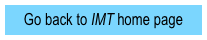Tutorials on phase space diagrams

Phase space diagrams:  Simple harmonic
motionPretest (.pdf)

Tutorial (.pdf)

Homework (.pdf)

Exam questions (.docx)

Instructor notes (.pdf)

All materials (~1MB .zip,

.docx and .pdf)

Tutorial title

Emphasis (what do students do?)

Gain familiarity with phase space diagrams

Use energy conservation to deduce shape and time-evolution of phase space trajectories

Explore effects of varying mass, spring constant, amplitude, and initial conditions

Phase space diagrams:  Damped harmonic motionContact information for co-PIs

Department of Physics

Grand Valley State University

Allendale, MI 49401

Tel.:  616-331-2524

FAX:  616-331-3740

Email:  ambroseb@gvsu.edu

Michael C. Wittmann

Department of Physics and Astronomy

5709 Bennett Hall

University of Maine

Orono, ME  04401-5709

Tel.:  207-581-1237

FAX:  207-581-3410

Email:  wittmann@umit.maine.edu

Reason semi-quantitatively about shape of phase space trajectories (e.g., ratio of successive amplitudes, axis crossings)

Distinguish between phase space plots of critically damped and overdamped oscillatorsPhase space diagrams:  Self-limiting oscillators

Pretest (.pdf)

Tutorial (.pdf)

Homework (.pdf)

Instructor notes (.pdf)

All materials (~1MB .zip,

.docx and .pdf)

Analyze qualitatively behavior of selected non-linear oscillators

Analyze and construct qualitatively correct phase space plots for (weakly damped) self-limiting oscillators

Pretest (.pdf)

Tutorial (.pdf)

Homework (.pdf)

Exam questions (.docx)

Instructor notes (.pdf)

All materials (~1MB .zip,

.docx and .pdf)

Sponsored in part by NSF grants DUE-0441426 and DUE-0442388Intermediate Mechanics Tutorials are modeled after:

1. Tutorials in Introductory Physics, L.C. McDermott P.S. Shaffer, and the Physics Education Group at the University of Washington (Prentice Hall, 2002)

1. Activity-Based Tutorials, M.C. Wittmann, R.N. Steinberg, E.F. Redish, and the University of Maryland Physics Education Research Group (Wiley, 2004 and 2005)